Learn All Concepts of Chapter 3 Class 12 Matrices - FREE. Check - Matrices Class 12 - Full video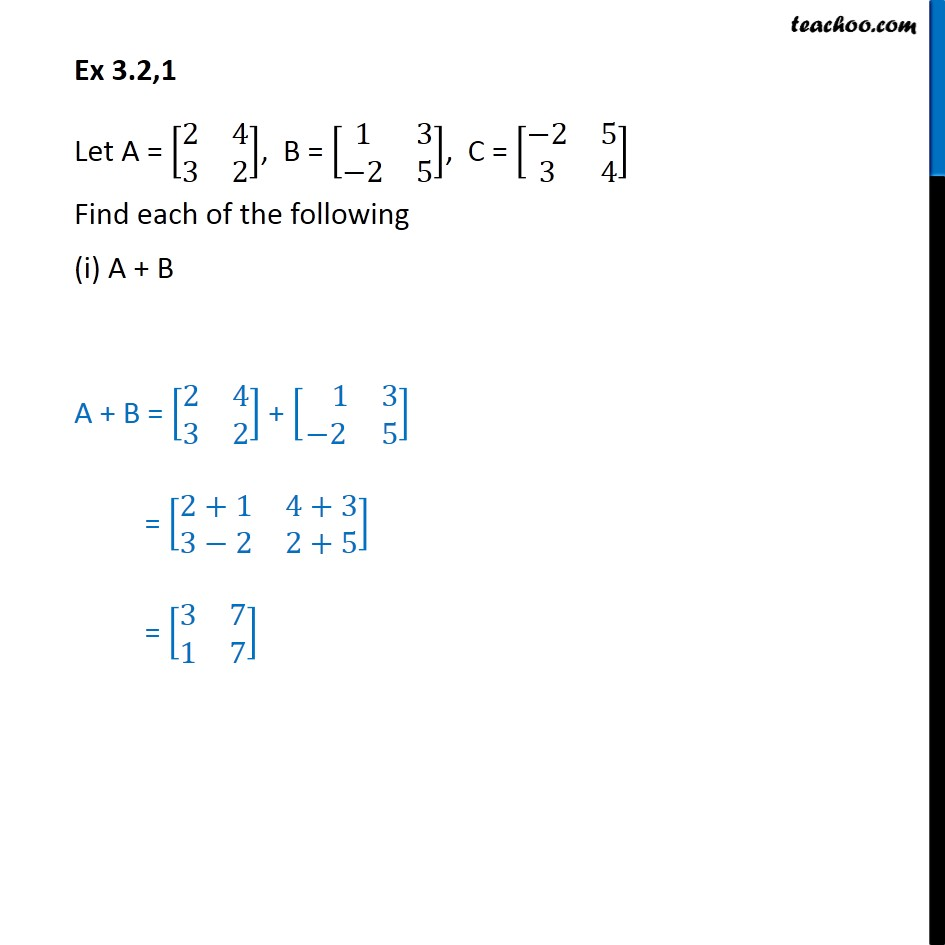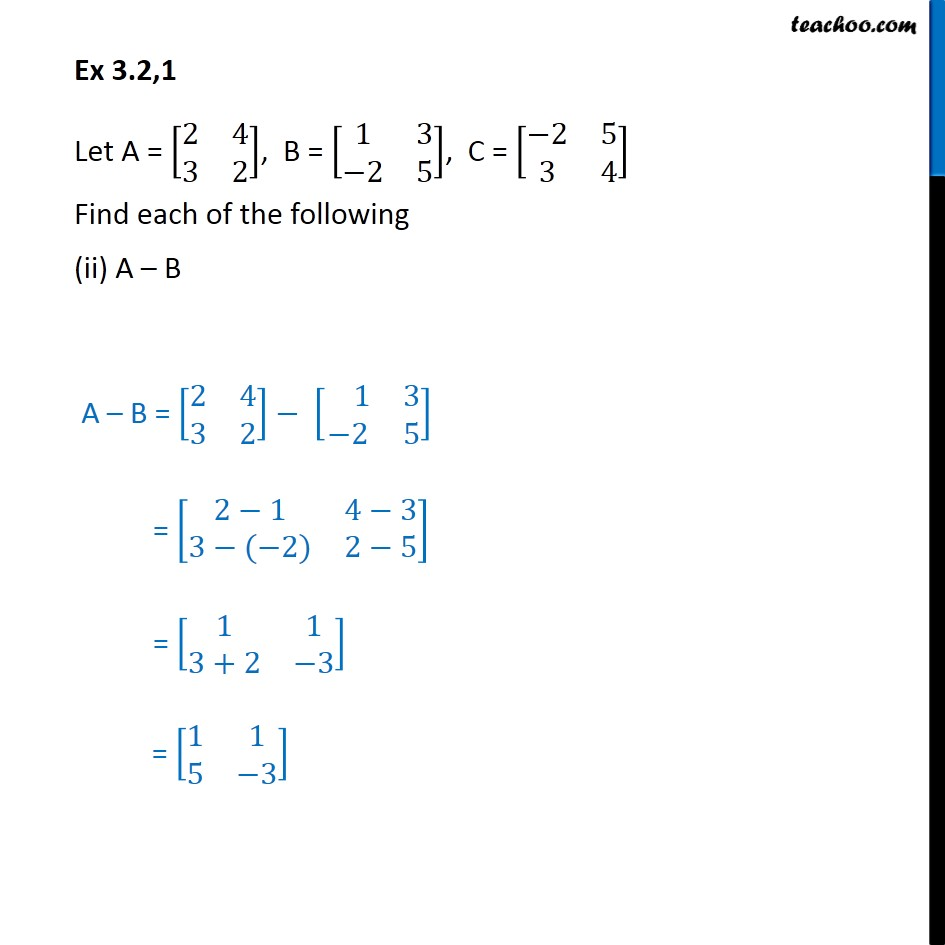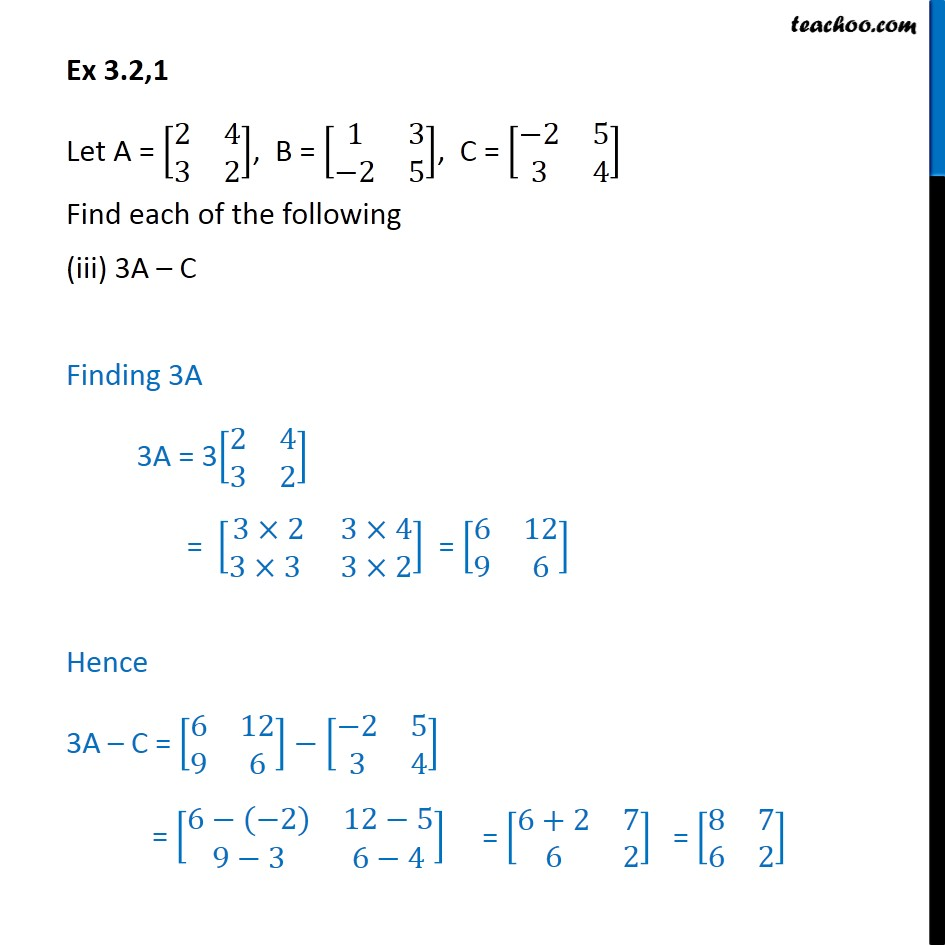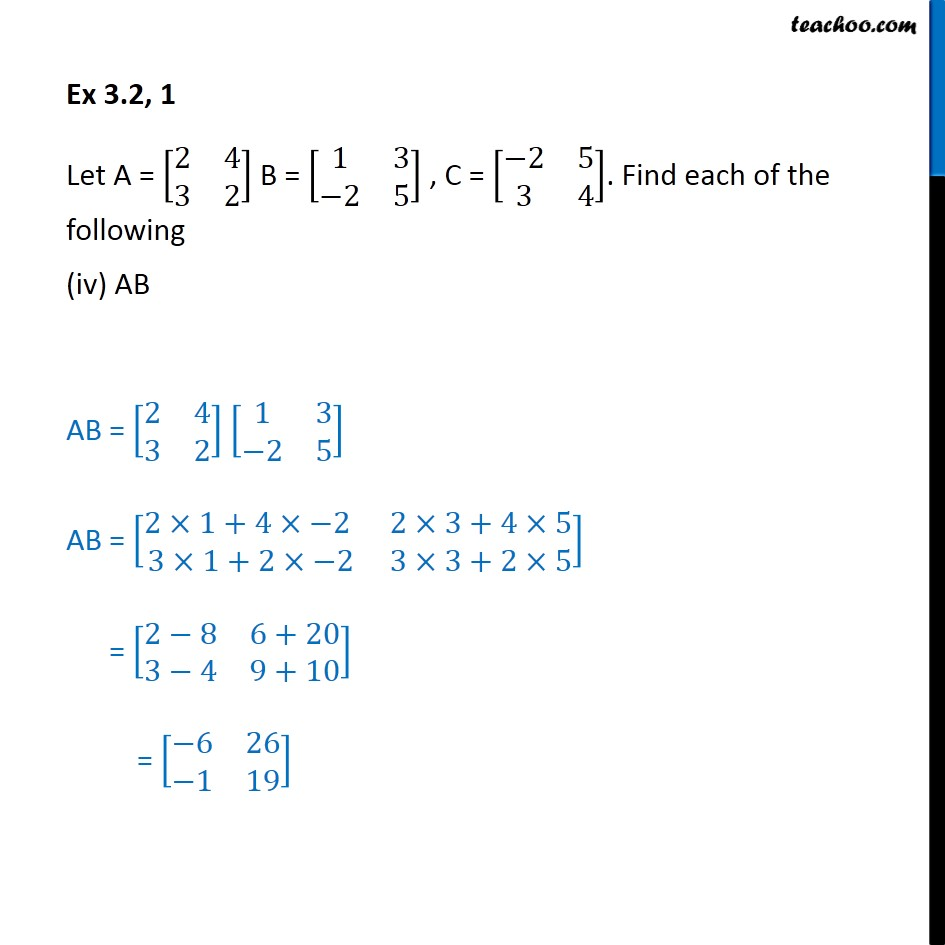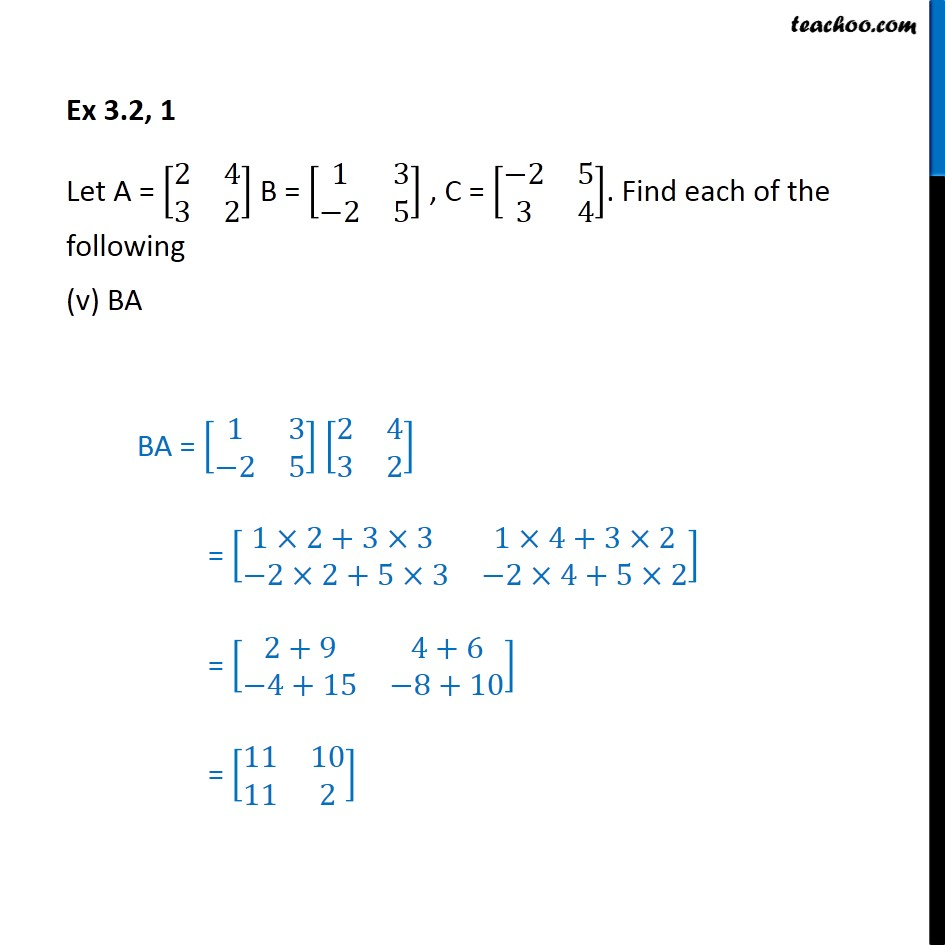1. Chapter 3 Class 12 Matrices
2. Serial order wise
3. Ex 3.2

Transcript

Ex 3.2,1 Let A = [ 8(2&4@3&2)], B = [ 8(1&3@ 2&5)], C = [ 8( 2&5@3&4)] Find each of the following (i) A + B A + B = [ 8(2&4@3&2)] + [ 8( 1&3@ 2&5)] = [ 8(2+1&4+3@3 2&2+5)] = [ 8(3&7@1&7)] Ex 3.2,1 Let A = [ 8(2&4@3&2)], B = [ 8(1&3@ 2&5)], C = [ 8( 2&5@3&4)] Find each of the following (ii) A B A B = [ 8(2&4@3&2)] [ 8( 1&3@ 2&5)] = [ 8(2 1&4 3@3 ( 2)&2 5)] = [ 8(1&1@3+2& 3)] = [ 8(1&1@5& 3)] Ex 3.2,1 Let A = [ 8(2&4@3&2)], B = [ 8(1&3@ 2&5)], C = [ 8( 2&5@3&4)] Find each of the following 3A C Finding 3A 3A = 3[ 8(2&4@3&2)] = [ 8(3 2&3 4@3 3 &3 2)] = [ 8(6&12@9&6)] Hence 3A C = [ 8(6&12@9&6)] 7 [ 8( 2&5@3&4)] = [ 8(6 ( 2)&12 5@9 3&6 4)] Ex 3.2, 1 Let A = [ 8(2&4@3&2)] B = [ 8(1&3@ 2&5)] , C = [ 8( 2&5@3&4)]. Find each of the following (iv) AB AB = [ 8(2&4@3&2)] [ 8(1&3@ 2&5)] AB = [ 8(2 1+4 2 &2 3+4 5@3 1+2 2&3 3+2 5)] = [ 8(2 8&6+20@3 4&9+10)] = [ 8( 6&26@ 1&19)] Ex 3.2, 1 Let A = [ 8(2&4@3&2)] B = [ 8(1&3@ 2&5)] , C = [ 8( 2&5@3&4)]. Find each of the following (v) BA BA = [ 8(1&3@ 2&5)] [ 8(2&4@3&2)] = [ 8(1 2+3 3 &1 4+3 2@ 2 2+5 3& 2 4+5 2)] = [ 8(2+9&4+6@ 4+15& 8+10)] = [ 8(11&10@11&2)]

Ex 3.2

Chapter 3 Class 12 Matrices
Serial order wise